Next: Bayesian statistics Up: Nonlinear Switching State-Space Models Previous: Prediction algorithms   Contents

# Bayesian methods for data analysis

Inclusion of the effects of noise into the model of a time series leads to the world of statistics -- it is no longer possible to talk about exact events, only their probabilities.

The Bayesian framework offers the mathematically soundest basis for doing statistical work. In this chapter, a brief review of the most important results and tools of the field is presented.

Section 3.1 concentrates on the basic ideas of Bayesian statistics. Unfortunately, exact application of those methods is usually not possible. Therefore Section 3.2 discusses some practical approximation methods that allow getting reasonably good results with limited computational resources. The learning algorithms presented in this work are based on the approximation method called ensemble learning, which is presented in Section 3.3.

This chapter contains many formulas involving probabilities. The notation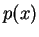is used for both probability of a discrete event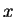and the value of the probability density function (pdf) of a continuous variable at, depending on whatis. All the theoretical results presented apply equally to both cases, at least when integration over a discrete variable is interpreted in the Lebesgue sense as summation.

Some authors use subscripts to separate different pdfs but here they are omitted to simplify the notation. All pdfs are identified only by the argument of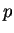.

Two important probability distributions, the Gaussian or normal distribution and the Dirichlet distribution are presented in Appendix A. The notation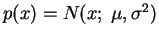is used to denote thatis normally distributed with mean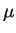and variance.

SubsectionsNext: Bayesian statistics Up: Nonlinear Switching State-Space Models Previous: Prediction algorithms   Contents
Antti Honkela 2001-05-30